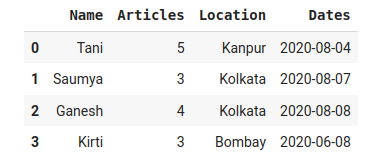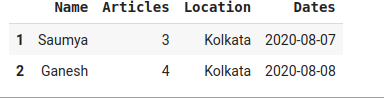Related Articles

# Select Pandas dataframe rows between two dates

• Difficulty Level : Expert
• Last Updated : 24 Feb, 2021

Prerequisites: pandas

Pandas is an open-source library that is built on top of NumPy library. It is a Python package that offers various data structures and operations for manipulating numerical data and time series. It is mainly popular for importing and analyzing data much easier. Pandas is fast and it has high-performance & productivity for users.

This article focuses on getting selected pandas data frame rows between two dates. We can do this by using a filter.

Dates can be represented initially in several ways :

• string
• np.datetime64
• datetime.datetime

To manipulate dates in pandas, we use the pd.to_datetime() function in pandas to convert different date representations to datetime64[ns] format.

Syntax: pandas.to_datetime(arg, errors=’raise’, dayfirst=False, yearfirst=False, utc=None, box=True, format=None, exact=True, unit=None, infer_datetime_format=False, origin=’unix’, cache=False)

Parameters:

• arg: An integer, string, float, list or dict object to convert in to Date time object.
• dayfirst: Boolean value, places day first if True.
• yearfirst: Boolean value, places year first if True.
• utc: Boolean value, Returns time in UTC if True.
• format: String input to tell position of day, month and year.

### Approach

• Import module
• Create dataframe
• Convert the dates column to datetime64[ns] data type
• Define a start date and end date.
• Use a filter to display the updated dataframe and store it.
• Display dataframe

Example: Original dataframe

## Python3

 `import` `pandas as pd``data ``=` `{``'Name'``: [``'Tani'``, ``'Saumya'``,``                 ``'Ganesh'``, ``'Kirti'``],`` ` `        ``'Articles'``: [``5``, ``3``, ``4``, ``3``],`` ` `        ``'Location'``: [``'Kanpur'``, ``'Kolkata'``,``                     ``'Kolkata'``, ``'Bombay'``],``        ``'Dates'``: [``'2020-08-04'``, ``'2020-08-07'``, ``'2020-08-08'``, ``'2020-06-08'``]}`` ` `# Create DataFrame``df ``=` `pd.DataFrame(data)``display(df)`

Output:Example: Selecting data frame rows between two rows

## Python3

 `import` `pandas as pd``data ``=` `{``'Name'``: [``'Tani'``, ``'Saumya'``,``                 ``'Ganesh'``, ``'Kirti'``],`` ` `        ``'Articles'``: [``5``, ``3``, ``4``, ``3``],`` ` `        ``'Location'``: [``'Kanpur'``, ``'Kolkata'``,``                     ``'Kolkata'``, ``'Bombay'``],``        ``'Dates'``: [``'2020-08-04'``, ``'2020-08-07'``, ``'2020-08-08'``, ``'2020-06-08'``]}`` ` `# Create DataFrame``df ``=` `pd.DataFrame(data)``start_date ``=` `'2020-08-05'``end_date ``=` `'2020-08-08'``mask ``=` `(df[``'Dates'``] > start_date) & (df[``'Dates'``] <``=` `end_date)`` ` `df ``=` `df.loc[mask]``display(df)`

Output:Attention geek! Strengthen your foundations with the Python Programming Foundation Course and learn the basics.

To begin with, your interview preparations Enhance your Data Structures concepts with the Python DS Course. And to begin with your Machine Learning Journey, join the Machine Learning – Basic Level Course

My Personal Notes arrow_drop_up